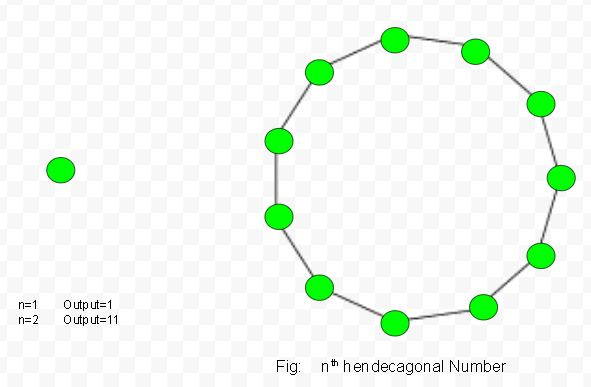# Hendecagonal number

Given a number n, the task is to find the nth Hendecagonal number.
A Hendecagonal number is a figurate number that extends the concept of triangular and square numbers to the decagon (Eleven -sided polygon). The nth hendecagonal number counts the number of dots in a pattern of n nested decagons, all sharing a common corner, where the ith hendecagon in the pattern has sides made of i dots spaced one unit apart from each other.

Examples:

Input : 2
Output :11

Input :6
Output :141Formula for nth hendecagonal number :## C++

 // C++ program to find nth  // Hendecagonal number  #include  using namespace std;     // Function to find  // Hendecagonal number  int hendecagonal_num(int n)  {      // Formula to calculate nth      // Hendecagonal number      return (9 * n * n - 7 * n) / 2;  }     // Driver Code  int main()  {      int n = 3;      cout << n << "rd Hendecagonal number: ";      cout << hendecagonal_num(n);      cout << endl;      n = 10;      cout << n << "th Hendecagonal number: ";      cout << hendecagonal_num(n);         return 0;  }

## Java

 // Java program to find nth  // Hendecagonal number  import java.io.*;     class GFG  {         // Function to find  // Hendecagonal number  static int hendecagonal_num(int n)  {      // Formula to calculate nth      // Hendecagonal number      return (9 * n * n -               7 * n) / 2;  }     // Driver Code  public static void main (String[] args)  {  int n = 3;  System.out.print(n + "rd Hendecagonal " +                               "number: ");  System.out.println(hendecagonal_num(n));     n = 10;  System.out.print(n + "th Hendecagonal " +                                "number: ");  System.out.println(hendecagonal_num(n));  }  }     // This code is contributed by ajit

## Python3

 # Program to find nth  # Hendecagonal number     # Function of Hendecagonal   # number   def hendecagonal_num(n) :             # Formula to calculate nth      # Hendecagonal number &      # return it into main function.             return (9 * n * n -             7 * n) // 2    # Driver Code  if __name__ == '__main__' :                 n = 3     print(n,"rd Hendecagonal number : " ,                       hendecagonal_num(n))         n = 10     print(n,"th Hendecagonal number : " ,                       hendecagonal_num(n))     # This code is contributed by ajit

## C#

 // C# program to find nth  // Hendecagonal number  using System;     class GFG  {  // Function to find  // Hendecagonal number  static int hendecagonal_num(int n)  {      // Formula to calculate nth      // Hendecagonal number      return (9 * n * n - 7 * n) / 2;  }     // Driver Code  static public void Main ()  {      int n = 3;      Console.Write(n +                    "rd Hendecagonal number: ");      Console.WriteLine( hendecagonal_num(n));         n = 10;      Console.Write(n +                    "th Hendecagonal number: ");      Console.WriteLine( hendecagonal_num(n));      }  }     // This code is contributed by aj_36

## PHP

 

Output :

3th Hendecagonal number: 30
10th Hendecagonal number: 415


Attention reader! Don’t stop learning now. Get hold of all the important DSA concepts with the DSA Self Paced Course at a student-friendly price and become industry ready.

My Personal Notes arrow_drop_upCheck out this Author's contributed articles.

If you like GeeksforGeeks and would like to contribute, you can also write an article using contribute.geeksforgeeks.org or mail your article to contribute@geeksforgeeks.org. See your article appearing on the GeeksforGeeks main page and help other Geeks.

Please Improve this article if you find anything incorrect by clicking on the "Improve Article" button below.

Improved By : jit_t

Article Tags :
Practice Tags :

Be the First to upvote.

Please write to us at contribute@geeksforgeeks.org to report any issue with the above content.